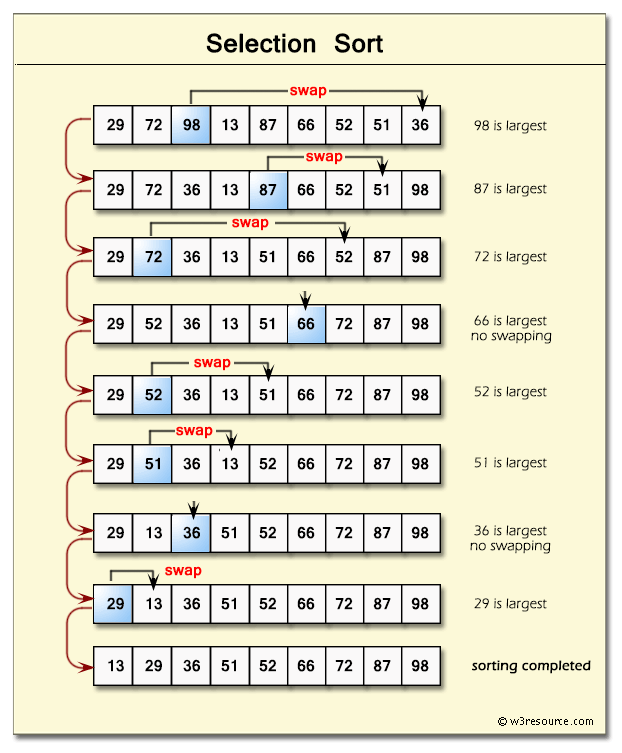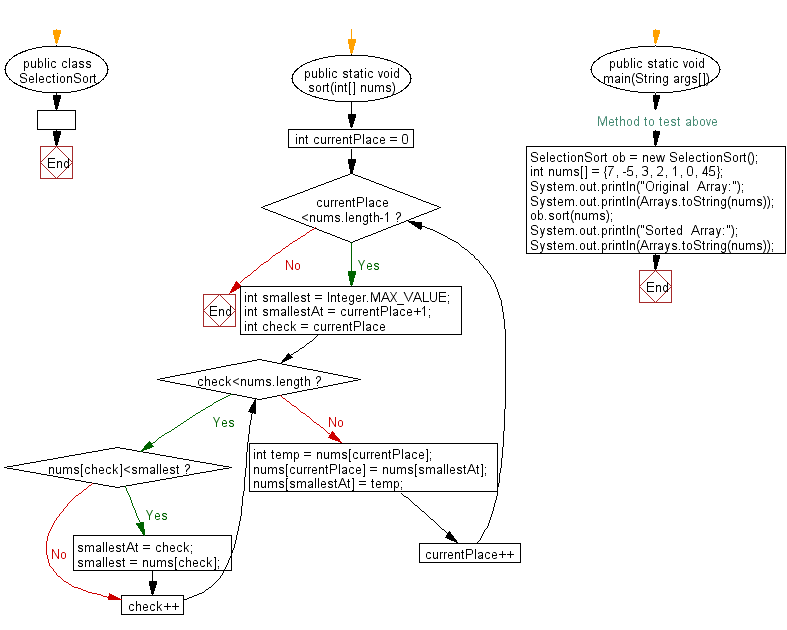﻿ Java exercises: Selection Sort Algorithm - w3resource# Java Exercises: Selection Sort Algorithm

## Java Sorting Algorithm: Exercise-6 with Solution

Write a Java program to sort an array of given integers using Selection Sort Algorithm.

According to Wikipedia “In computer science, selection sort is a sorting algorithm, specifically an in-place comparison sort. It has O(n2) time complexity, making it inefficient on large lists, and generally performs worse than the similar insertion sort”.

Note:
a) To find maximum of elements
b) To swap two elements

Pictorial presentation - Selection search algorithm :Sample Solution:

Java Code:

``````import  java.util.Arrays;
public class  SelectionSort {
public static void sort(int[] nums)
{
for(int currentPlace = 0;currentPlace<nums.length-1;currentPlace++){
int smallest =  Integer.MAX_VALUE;
int smallestAt =  currentPlace+1;
for(int check =  currentPlace; check<nums.length;check++){
if(nums[check]<smallest){
smallestAt  = check;
smallest  = nums[check];
}
}
int temp =  nums[currentPlace];
nums[currentPlace]  = nums[smallestAt];
nums[smallestAt]  = temp;
}
}
// Method to test above
public static void main(String args[])
{
SelectionSort ob = new SelectionSort();
int nums[] = {7, -5, 3, 2, 1, 0, 45};
System.out.println("Original  Array:");
System.out.println(Arrays.toString(nums));
ob.sort(nums);
System.out.println("Sorted  Array:");
System.out.println(Arrays.toString(nums));
}
}
``````

Sample Output:

```Original  Array:
[7, -5, 3, 2, 1, 0, 45]
Sorted  Array:
[-5, 0, 1, 2, 3, 7, 45]
```

Flowchart:Java Code Editor:

Contribute your code and comments through Disqus.

What is the difficulty level of this exercise?

﻿